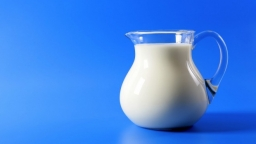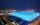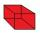# Cube-shaped box

The cube-shaped box is filled to the brim with 2 liters of milk. Calculate the edge and surface of the box.

a =  12.5992 cm
S =  952.4406 cm2

### Step-by-step explanation:Did you find an error or inaccuracy? Feel free to write us. Thank you!Tips to related online calculators
Do you know the volume and unit volume, and want to convert volume units?

## Related math problems and questions:

• The cubeThe cube has a surface area of 216 dm2. Calculate: a) the content of one wall, b) edge length, c) cube volume.
• The cubeThe cube has a surface area of 486 m ^ 2. Calculate its volume.
• Cube surface and volumeFind the surface of the cube with a volume of 27 dm3.
• Area to volumeIf the surface area of a cube is 486, find its volume.
• Cube surfce2volumeCalculate the volume of the cube if its surface is 150 cm2.
• Cube V2SThe volume of the cube is 27 dm cubic. Calculate the surface of the cube.
• Cube 6The surface area of one wall cube is 1600 cm square. How many liters of water can fit into the cube?
• TerezaThe cube has an area of base 256 mm2. Calculate the edge length, volume, and area of its surface.
• Length of the edgeFind the length of the edge of a cube with a cm2 surface and a volume in cm3 expressed by the same number.
• Three cubesTwo cube-shaped boxes with edges a = 70 cm; b = 90 cm must be replaced by one cube-shaped box. What will be its edge?
• Special cubeCalculate the edge of cube, if its surface and its volume is numerically equal number.
• Cube-shaped containerThe cube-shaped container has a height of 52 cm and a square base. The container was filled to the brim with water, then we immersed a metal cube in it, which caused 2.7 l of water to flow out of the container. After removing the cube from the water, the
• ContainerThe container-shaped box with internal dimensions of 3.9 m, 3.25 m and 2.6 m was completely filled with goods in the same cubic boxes. How long edge could this box have?
• The cubeThe cube has a surface of 600 cm2. What is its volume?
• Chemical parisonThe blown parison (with shape of a sphere) have a volume 1.5 liters. What is its surface?
• Cuboid to cubeA cuboid with dimensions of 9 cm, 6 cm, and 4 cm has the same volume as a cube. Calculate the surface of this cube.
• Cube 6Volume of the cube is 216 cm3, calculate its surface area.